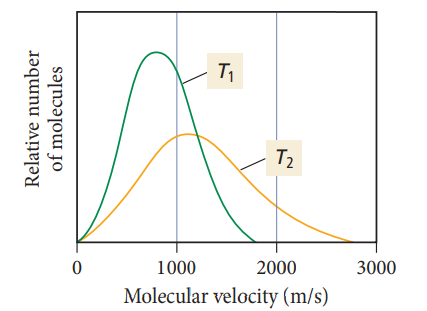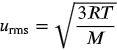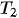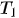1
204
views
60
Problem

# Problem 60

## Chapter 5: GasesTextbook ExpertVerified Tutor
26 Oct 2021

#### Given information

The graph of the molecular velocity of the same molecule at two different temperatures is given as -:#### Step-by-step explanation

Step 1.

Root mean square speed formula -:As per the given formula of average velocity of gas molecules, the root mean square velocity is directly proportional to the temperature. As the molecular velocity at temperatureis higher than that of. Therefore, the temperatureis higher than.